/

### Coherent Risk Measures

A risk measure,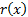is defined by Artzner et al. (1999) to be coherent if it satisfies the following 4 axioms:

(a)    Subadditivity: for any pair of loss variables,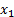and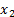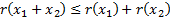(b)   Monotonicity: if, for all states of the world,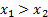then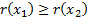(c)    Homogeneity: for any constant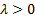and random loss variable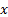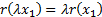(d)   Translational invariance: for any constant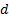and random loss variable x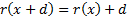Artzner et al. (1999) also showed that a risk measure is coherent if and only if there is a family,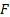, of probability measures,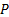, such that: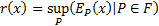Sometimes the easiest way of proving that a risk measure is coherent is to prove each of the four axioms are satisfied, at other times it is easiest to show that it may be expressed in this supremum form.

For example TVaR can be shown to be coherent by defining a set of probability measures that place equal probability on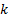realisations whereis the smallest integer such that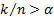. TVaR is then the maximum expected value of losses over this family of distributions.

In contrast, VaR is coherent only for a limited class of distributions, including multi-variate Normal (i.e. Gaussian) distributions (for proof see here) and more generally for elliptical distributions.